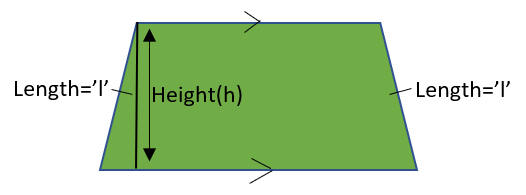GeeksforGeeks App
Open AppBrowser
Continue

# Isosceles Trapezoid Formulas

Trapezoid is a quadrilateral in which a pair of opposite sides are parallel. It is also known as Trapezium. There are three types of Trapezoids and Isosceles Trapezoid is one of its type. The types of Trapezoids are:

1. Right Trapezoid
2. Isosceles Trapezoid
3. Scalene Trapezoid

### Isosceles Trapezoid

Isosceles Trapezoid is a trapezium with congruent base angles and congruent non parallel sides. A trapezium is called an Isosceles trapezoid when two opposite sides (Bases) are parallel and the other two sides (legs) are of the same length.Area and Perimeters are the formulas of Isosceles Trapezoid.

### Area of Isosceles Trapezoid

The area of the Isosceles Trapezoid can be calculated by adding the lengths of two parallel sides (bases) and dividing this by 2 and multiplying the result with the height of the trapezium to get the area. The area formula is given by-

Area = ((a+b)/2) × h

Where,

a, b are the length of parallel sides

and h is the height.

To get more understanding let’s solve a few examples

### Sample Problems on Area of Isosceles Trapezium

Question 1: What is the area of isosceles trapezium if the length of parallel sides are 7cm, 5cm and height is 4cm.

Solution:

Given

Length of parallel sides (a) = 7cm, b = 5cm

Height (h) = 4cm

Area = ((a + b)/2) × h

= ((7 + 5)/2) × 4

= (12/2) × 4

= 6 × 4

= 24 cm2

Area of given isosceles trapezoid is 24cm2.

Question 2: Find the height of isosceles trapezium if the length of parallel sides are 6cm, 4cm and area is 24cm2.

Solution:

Given

Length of parallel sides (a) = 6cm, b = 4cm

Area = 24cm2

Area = ((a+b)/2) × h

24 = ((6+4)/2) × h

24 = (10/2) × h

24 = 5 × h

h = 24/5

= 4.8cm

So from given area, base lengths the height of an isosceles trapezium is 4.8cm

### Perimeter of Isosceles Trapezoid

The perimeter of an isosceles trapezoid can be calculated by adding all sides of the trapezoid. The perimeter formula is given by-

Perimeter = a+b+c+d

Where,

a,b are lengths of two parallel sides

c,d are length of two unparallel sides

Note: For isosceles trapezoid c = d (Unparallel side lengths are equal)

Let’s look into a few examples to get more understanding.

### Sample Problems on Perimeter of Isosceles Trapezoid

Question 1: What is the perimeter of an isosceles trapezoid if the length of sides are 7cm, 5cm, 3cm, 3cm.

Solution:

Given,

Length of parallel sides (a) = 7cm, (b) = 5cm

Length of un parallel sides (c) = 3cm, (d) = 3cm

Perimeter = a + b + c  + d

= 7+5+3+3

= 18cm

So perimeter of given isosceles trapezium is 18cm.

Question 2: What is the perimeter of an isosceles trapezoid if the length of parallel sides are 8cm, 4cm and length of sides of equal lengths 2cm.

Solution:

Given,

Length of parallel sides (bases) (a) = 8cm, (b) = 4cm

Length of un parallel sides (legs) (c) = 2cm, (d) = 2cm

Perimeter = a + b + c  + d

= 8 + 4 + 2 + 2

= 16cm

So perimeter of given isosceles trapezium is 16cm.

Question 3: What is the area and perimeter of an isosceles trapezium with base lengths are 3cm, 6cm and the length of the other 2 sides which are equal in length is 2.5cm and height is 1.5cm.

Solution:

Given

Length of bases (a) = 6cm, (b) = 3cm

Length of legs i.e., sides with equal lengths (c) = 2.5cm, (d) = 2.5cm

Height (h) = 1.5cm

Area =((a+b)/2) × h

= ((6+3)/2) × 1.5

= (9/2) × 1.5

= 4.5 × 1.5

= 6.75cm2

Perimeter = a + b + c + d

= 6 + 3 + 2.5 + 2.5

= 14cm

So for the given data, Area is 6.75cm2 & perimeter is 14cm.

My Personal Notes arrow_drop_up
Related Tutorials